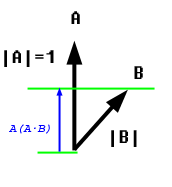Gilbert Strang and Linear Alzebra

Home page of Gilbert Strang at MIT

```Two vectors lie in a plane
Each at a varied incline
As they dance together
There isn't a square
That is uncovered in the infinite plane
```

When two vectors are added with different weights in a plane the resultant vecctor can take any point on that plane.

The visual geometric proof is very simple and elegant.

U keep one vector constant and change the size of the other vector from negative infinity to positive infinity.

That traverses a line. Now the other vector can move the line from one end to the other covering all space.

AX: is a way to linearly combine all columns into a resulting column

X is a row of weigths.

``````
Column Vectors
Row vectors
Elimination Matrices
Sub Spaces
Least Squares
Determinants
Inverses
Pivots
Singular
Eigen Values
``````

Eigenvalues and eigenvectors are an astonishing way to understand a square matrix - GS

And the Fast Fourier Transform (multiplying quickly by F and F-1) is a revolutionary algorithm. - GS

Calculus is mostly about one special operation (the derivative) and its inverse (the integral). Of course I admit that calculus could be important .... But so many applications of mathematics are discrete rather than continuous, digital rather than analog. The century of data has begun! You will find a light-hearted essay called "Too Much Calculus" on my website. The truth is that vectors and matrices have become the language to know.

A key goal is learning to "read" a matrix. You need to see the meaning in the numbers. This is really the essence of mathematics-patterns and their meaning.

The greatest encouragement of all is the feeling that you are doing something worthwhile with your life - GS

The heart of linear algebra is in two operations-both with vectors - GS

The entire x-y plane and every point on that place can be seen as a linear collection vectors on the X and the Y axis

what does a dot product of 2 vectors truly represent?

Search for: what does a dot product of 2 vectors truly represent?

``````
A.B = |A|.|B|.cos(angle)

Or

A.B = |A|.[|B|.cos(angle)]

that means
``````

project vector B on to vector A and see what is its length. Then multiply the length of A with it. This means the magnitude of B is reduced by its angle and then see what their multiplier of the new adjusted magnitudes are.Taken from

Project it first and then multiply with the other length

Better yet: http://math.oregonstate.edu/bridge/papers/dot+cross.pdf

it is fundamentally a projection

Pivots in elimination cannot be zero

The matrix left behind after eliminating all variables except the last one.

A determinant is the multiplication of all pivots of the U matrix

Most of the elimination in linear equations is SQL like operations on row vectors, or rows

multiple row 3 with column 4 of the respective multiplying matrices :)

Each elimination step is done by a separate elimination matrix

where U is the upper triangular

Fun part is the big E matrix above tells us the whole story in a single matrix: That ought to be NICE

A permutation matrix swaps two rows of a matrix

Kind of the flipped identity matrix on the left

Beautiful: Every column of a right side matrix produce an independent column that is a combination of left hand side matrix columns

A permutation matrix exchanges rows of a matrix

A permutation matrix is an identity matrix with its rows exchanged

``````
P - The permutation matrix
A - The augmented matrix of linear equations
L - The lower triangle matrix via elimination
U - The upper triangular matrix of what is left
``````

Permutation matrix is an identity matrix with reordered rows - GS

There are n! ways of reordering rows in a matrix

``````
P(-1) = P(T)

P Inverse = P Transposed.
``````

In other words if you want to find an inverse of a permutation matrix P, just transpose it.

P.P(T) = I as in P.P(-1) = I

``````
A is symmetric if

A = A(T)
``````
``````
[R.R(T)](T) = [R(T)(T).R(T)]

= [R.R(T)]

See we ended up with the same
``````

where a set of vectors when combined linearly (add, subtract, multiply) still stay in the same selected space.

So an XY plane is a vector space.

A quadrant is not a vector space because a combination of two vectors will yield vectors outside of that.

Any line that goes through the origin is a vector space because the vectors on that line can dance together and never cross that line :)

However a line that doesn't go through the origin will shoot that line above or below based on a multiplier and thus escaping the original space where the vector came from

1, Entire plane

2. Any line through zero

3. The origin itself

A line in R2 is not R1 because it has 2 components while R1 has only 1 component

Take its columns

Consider each column a vector

And the column space will come from all linear combinations of those vectors. All linear combinations mind you!!